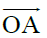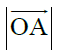# Find the position vector of a point A in space such`
Question:

Find the position vector of a point A in space such thatis inclined at 60º to OX and at 45° to OY and= 10 units.

Solution:

We know that, cos2 α + cos2 β + cos2 γ = 1

cos2 60o + cos2 45o + cos2 γ = 1

(1/2)2 + (1/√2)2 + cos2 γ = 1

¼ + ½ + cos2 γ = 1

cos2 γ = 1 – ¾ = ¼

So, cos γ = ± ½ ⇒ cos γ = ½ [Rejecting cos γ = – ½, as γ < 90o]

Now,

$\overrightarrow{\mathrm{OA}}=|\overrightarrow{\mathrm{OA}}|\left(\frac{1}{2} \hat{i}+\frac{1}{\sqrt{2}} \hat{j}+\frac{1}{2} \hat{k}\right)=10\left(\frac{1}{2} \hat{i}+\frac{1}{\sqrt{2}} \hat{j}+\frac{1}{2} \hat{k}\right)$

$=5 \hat{i}+5 \sqrt{2} \hat{j}+5 \hat{k}$

Thus, the position vector of $\mathrm{A}$ is $(5 \hat{i}+5 \sqrt{2} \hat{j}+5 \hat{k})$.